International Journal of Systems Science and Applied Mathematics
Volume 1, Issue 4, November 2016, Pages: 86-90

Performance Evaluation of Empirical Rain Rate Models for Computing Rain Attenuation

Ezenugu Isaac Amazuilo1, *, Nseobong. I. Okpura2, Enyenihi Henry Johnson3

1Department of Electrical Engineering, Imo State University (IMSU), Owerri, Nigeria

2Department of Electrical/Electronic and Computer Engineering, University of Uyo, Akwa Ibom, Nigeria

3Department of Electrical/Electronic Engineering, Akwa Ibom State University, Mkpat Enin, Nigeria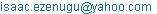(E. I. Amazuilo)

*Corresponding author

Ezenugu Isaac Amazuilo, Nseobong. I. Okpura, Enyenihi Henry Johnson. Performance Evaluation of Empirical Rain Rate Models for Computing Rain Attenuation. International Journal of Systems Science and Applied Mathematics. Vol. 1, No. 4, 2016, pp. 86-90. doi: 10.11648/j.ijssam.20160104.19

Received: October 16, 2016; Accepted: December 28, 2016; Published: January 21, 2017

Abstract: When computing rain attenuation with the International Telecommunication Union (ITU) model the rain rate is normally needed. However, only limited rain rate data are published by ITU for the various regions all over the globe. In order to obtain the missing rain rate data for ITU rain attenuation model, two empirical models used to generate the rain rate data for the fifteen ITU rain zones are presented in this paper. The performance of the two empirical models (referred to as Model 1 and Model 2) is also presented. Specifically, two ITU rain zones (N and P) are considered and Model 1 is used for estimating the rain rate in rain zone P, whereas, Model 2 is used for estimating the rain rate in rain zone N. The rain attenuation computed based on the ITU rain rate generated from the empirical models and the rain attenuation computed from the actual ITU rain rata data are compared in terms of Root Mean Square Error (RMSE), coefficient of determination (r2) and prediction accuracy. According to the results, in respect of rain rate prediction for rain zone N, Model 2 has RMSE (3.491375), Coefficients of Determination (r2= 0.99816) and Prediction Accuracy (92.49319835%). Also, for rain zone N, with respect to the rain attenuation, Model 2 had RMSE of 0.792219, the Coefficients of Determination (r2) of 0.99855 and the Prediction Accuracy of 90.51054739%. Similarly, in respect of rain rate prediction for rain zone P, Model 1 has RMSE (4.924732), Coefficients of Determination (r2= 0.99798) and Prediction Accuracy (92.29421163%). In addition, with respect to the rain attenuation for rain zone P, Model 1 has RMSE is 1.276727, the Coefficients of Determination (r2) is 0.99812 and the Prediction Accuracy 90.31393492%. In all, the two prediction accuracies of the two empirical models are adjudged very good in predicting the rain rates. As such, the rain rates predicted by those models are suitable for computing the rain attenuations for the ITU rain zones.

Keywords: Rain Rate, Rain Attenuation, Empirical Models, ITU Rain Zone, RMSE, Coefficients of Determination, Prediction Accuracy

Contents

1. Introduction

According to experts, rain is the dominant propagation phenomena on wireless links with frequencies above 10 GHz . The impairment of signal due to rain depends, among other things, on rain rate, size of raindrops and rain drop density [1-4]. The International Telecommunication Union–Radiocommunication Sector (ITU-R) model was adjudged the most widely accepted internationally for the prediction of the effect of rain on communication systems [5-8]. Generally, specific attenuation, γR(dB/km) is obtained from the rain rate R (mm/hr) using the power law relationship [4-7,9,13]:

γR = kRα                     (1)

where α and k are coefficients that are dependent on polarization and frequency. Furthermore, the rain attenuation in dB is given as ;

AR = (γR)d                   (2)

where, d, is the effective path length; and γR, the specific attenuation, where, k and α, are frequency dependent constants.

According to ITU-R PN.837-1 recommendation, the world is split into 15 regions according to rain rates [14-15]. The rain rate data is normally given for a particular percentage of time exceeded. The data on the rain rate for different rain zones are published by ITU are for only seven different percentage of time exceeded. As such, for rain attenuation analysis requiring the rain rate for other percentage of time exceeded, models are developed to estimate the rain rates. The models are developed based on the available ITU rain rate data for different rain zones and percentage of time exceeded. The focus in this paper is to evaluate the effectiveness of using such model generated rain rate data in the computation of rain attenuation for the ITU rain zones. Specifically, two of such empirical models are considered in this paper and their application in the prediction of rain rate for two (2) ITU rain zones, namely, rain zone N and P are studied.

The earlier work in  used 15 different trendline model, one for each of the 15 ITU rain zones. The trendline models in  are more complex than the simplified two models presented in this paper. Also, the authors in  did not show the application of the rain rate predicted by such trendline models in the rain attenuation computation and how the computed rain attenuations differ from the rain attenuation computed from the actual ITU rain rate data.

Consequently, in this paper, first, the empirical rain rate models are used to estimate the rain rate for the rain zones and then the ITU rain attenuation model is used to compute the rain attenuation for the rain zones. Furthermore, the prediction efficiency of the models with respect to the rain rate and the rain attenuation are quantified in terms of Root Mean Square Error (RMSE), coefficient of determination (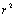) and the prediction accuracy.

2. Methodology

a    The ITU Rain Fade Model

For frequencies under 40 GHz and path lengths shorter than 60 km specific attenuation originating from rainfall is defined by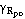in dB/km and modeled using the power-law equation as follows [9, 4]: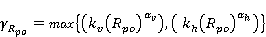(3)

where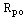is the rain rate exceeded for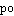%.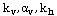and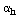are frequency dependent coefficients for vertical and horizontal polarization situations respectively [10, 12].

The rainfall rate,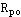has different values for each climate zone, also referred to as rain zone and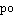is the Percentage outage time (or Percentage unavailability time) of the link. . Limited values offor the fifteen (15) ITU rain zones are provided in [4, 11]. However, in this paper, two empirical models are used to generate the values offor any given value of Po. The two empirical models are;

MODEL 1; Y =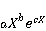(4)

MODEL 2; Y =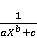(5)

where Y is the rain rate in mm/h, X (in %) is the percentage of time the rain rate is exceeded, and the values of the model coefficient (a, b, c) and constant (Kopt) are given in Table 1 and Table 2 for all the fifteen (15) ITU rain zones.

Model 1 is used for computing the rain rate for rain zone A, C, D, E, G, J, P and Q. On the other hand, Model 2 is used for computing the rain rate in rain zones B, F, H, K, L, M and N.

Table 1. Values For The Empirical Constants A, B and C and The Model Optimization Constant (Kopt) For Model 1 and The ITU Rain Zones Model 1 Applies To.

 MODEL 1 A b c Kopt A 1.261 -0.4148 -3.887 0.093228 C 2.387 -0.4148 -3.887 0.749839 D 3.976 -0.3416 -0.7553 0.161511 E 2.309 -0.4945 -1.877 0.234747 G 6.264 -0.3392 -0.8824 0.318893 J 13.71 -0.2027 -0.6551 0.762792 P 49.09 -0.2379 -2.091 3.741203 Q 53.91 -0.1668 -0.8625 1.118311

Table 2. Values For The Empirical Constants A, B and C and The Model Optimization Constant (Kopt) For Model 2 and The ITU Rain Zones Model 2 Applies To.

 MODEL 2 A b C Kopt B 1.392 0.6512 0.01577 -0.25442 F 0.6567 0.6953 0.007375 0.169725 H 0.4108 0.601 0.005593 -0.48374 K 0.397 0.6739 0.006244 -0.76367 L 0.3157 0.7038 0.004226 -0.52586 M 0.1787 0.6364 0.006129 -0.74685 N 0.1083 0.6198 0.004093 -2.72616

If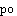is the Percentage outage time (or Percentage unavailability time) of the link and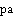is the Percentage availability time of the link. Then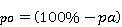(6)

Fundamentally, rain attenuation,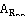(dB) is the product of specific rain attenuation,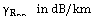and the propagation path length, d (km) between the transmitter and the receiver.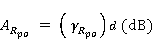(7)

b    Goodness of Fit Measures

The Coefficients of Determination (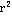): If n is the number of data items and we denote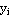as the observed values of the dependent variable,as its mean, and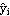as the fitted value, then the coefficient of determination is:(8)

Prediction Accuracy: The prediction accuracy (PA in %) is calculated as follows: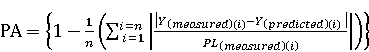(9)

Root Mean Square Error (RMSE): The RMSE is calculated as follows: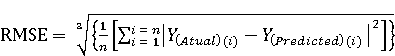(10)

where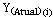is the actual rain rate given by ITU and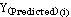is model predicted rain rate.

c     The Algorithm For Comparative Analysis of The Rain Attenuation Computed Based On The Actual ITU Rain Rate Data and The Empirical Model Generated ITU Rain Rate Data

1)     Input the operating frequency, (f) in GHz

2)     Input The Path Length (d) in Km

3)     Input the frequency constants for rain attenuation computation; (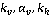and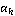)

4)     Input rain zone (RZ)

5)     Select model (MDL) based on Rain Zone

6)     Input model coefficients (a, b, c ) and the model constant (Kopt)

7)     Let Po(1) = 1; Po(2) = 0.3; Po(3) = 0.01; Po(4) = 0.03; Po(5) = 0.00 1; Po(6) = 0.003; Po(7) = 0.0001;

8)     For i = 1 to 7 Step 1

9)     Input Actual Rain Rate Data (ARRT(i) ) for rain zone RZ and percentage outage of Po(i)

10)     Compute rain attenuation AAR(i) with ARRT(i)

11)     Use Model MDL to compute Model Estimated Rain Rate Data (MRRT(i) ) for rain zone (RZ) and percentage outage of Po(i)

12)     Compute rain attenuation MAR(i) with MRRT(i) and the model for the given rain zone

13)     Next i

14)     Prepare table for Po(i), AAR(i) and MAR(i) where i = 1, 2, 3,…, 7

15)     Compute Root Mean Square Error (RMSE)

16)     Compute Coefficient of Determination (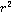)

17)     Compute Prediction Accuracy

18)     Plot Graph of AAR(i) and MAR(i) versus Po(i)

19)     End

3. Results and Discussion

The effectiveness of the empirical models in estimating the ITU rain data and hence in the computation of rain attenuation is demonstrated through sample computations of rain attenuations for a 10 GHz microwave link with a path length of 5 Km. The microwave link is considered is two ITU rain zones which are found in Nigeria, namely, rain zone N and P. The data for the rain attenuation computation are given in Table 3.

Table 3. The Input Data For The Rain Attenuation Computation For Rain Zones N and P.

 kh Ah Kv Av Frequency (f) in GHz Path Length (d) in Km 0.01217 1.2571 0.01129 1.2156 10 5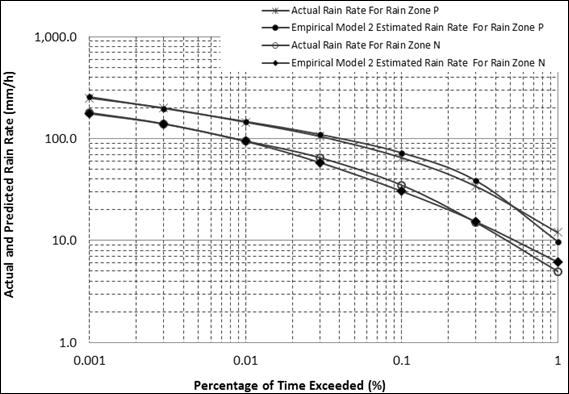Figure 1. Actual and Predicted Rain Rate (mm/h) Versus Percentage of Time Exceeded For ITU Rain Zones N and P.

The actual ITU rain rate for the rain zones N and P are given in Table 4 and Figure  1 along with the rain rates estimated by the empirical models. Specifically, Model 1 is used for estimating the rain rate in rain zone P, whereas, Model 2 is used for estimating the rain rate in rain zone N. The graph of the actual and predicted rain rate is shown in Figure  1 while the goodness of fit of the models’ prediction is given in Table 6. For rain zone N, Model 2 has RMSE (3.491375), Coefficients of Determination (= 0.99816) and Prediction Accuracy (92.49319835%). With coefficients of determination() being over 99% and also Prediction Accuracy over 92 %, it is obvious that the empirical model 2 can effectively estimate the rain rate for rain zone N.

Table 4. Actual Rain Rate and The Model Predicted Rain Rate For Rain Zone P.

 Po(i) Actual Rain Rate (mm/h) For Rain Zone P Empirical Model 2 Estimated Rain Rate (mm/h) For Rain Zone P Actual Rain Rate (mm/h) For Rain Zone N Empirical Model 2 Estimated Rain Rate (mm/h) For Rain Zone N 1 12 9.806936 5 6.166002 0.3 34 38.65145 15 15.29986 0.1 65 72.61945 35 30.49596 0.03 105 109.9212 65 58.15793 0.01 145 147.5257 95 94.0347 0.003 200 198.0362 140 139.0651 0.001 250 257.1285 180 176.1306

Similarly, in Table 6, for rain zone P, Model 1 has RMSE (4.924732), Coefficients of Determination (= 0.99798) and Prediction Accuracy (92.29421163%). With coefficients of determination () being over 99% and also Prediction Accuracy over 92%, it is obvious that the empirical model 1 can effectively estimate the rain rate for rain zone P.

The rain attenuation computed from the actual ITU rain rate and the model predicted ITU rain rate for rain zone N and P are given in Table 4 and Figure  2. The graph of the actual and predicted rain attenuations are shown in Figure  2 while the goodness of fit of the models’ prediction is given in Table 6. For rain zone N, Model 2, with respect to the rain attenuation, the RMSE is 0.792219, the Coefficients of Determination () is 0.99855 and the Prediction Accuracy 90.51054739%. With coefficients of determination () being over 99% and also Prediction Accuracy over 90 %, it is obvious that the empirical model 2 can effectively estimate the rain rate for computing the rain attenuation in rain zone N.

Table 5. Rain Attenuation Computed From Actual Rain Rate and The Model Predicted Rain Rate For Rain Zone N and P.

 Po(i) Rain Attenuation Based on Actual Rain Rate For Rain Zone P Rain Attenuation Based on Empirical Model 1 Estimated Rain Rate For Rain Zone P Rain Attenuation Based on Actual Rain Rate For Rain Zone N Rain Attenuation Based on Empirical Model 2 Estimated Rain Rate For Rain Zone N 1 1.383245694 1.073290155 0.4602 0.5989 0.3 5.122508989 6.018478293 1.8312 1.8773 0.1 11.56841775 13.29812016 5.3126 4.4679 0.03 21.13964308 22.39257756 11.5684 10.0589 0.01 31.71876711 32.41485984 18.6405 18.4027 0.003 47.5209802 46.93514885 30.35 30.0954 0.001 62.9087372 65.17190556 41.6259 40.5042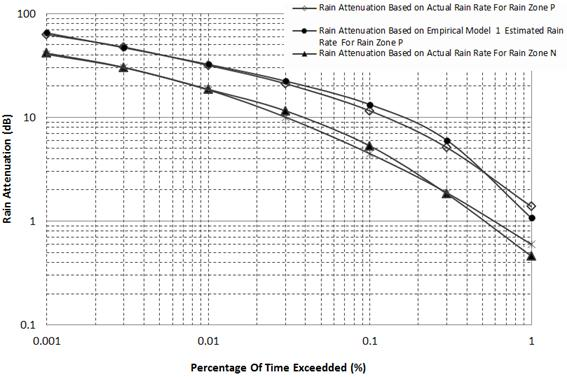Figure 2. Rain Attenuation computed from the Actual Rain Rate and the Model Predicted rain Rate For Rain Zone P and N versus Percentage of Time Exceeded.

Table 6. RMSE, Coefficients Of Determination (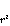) and Prediction Accuracy (%) Of The Models For The Two Rain Zones Considered.

 RMSE Coefficients of Determination (r-Squared): Prediction Accuracy (%) Rain Rate For Rain Zone N 3.491375 0.99816 92.49319835 Rain Attenuation For Rain Zone N 0.792219 0.99855 90.51054739 Rain Rate For Rain Zone P 4.924732 0.99798 92.29421163 Rain Attenuation For Rain Zone P 1.276727 0.99812 90.31393492

Similarly, in Table 6, for rain zone P, Model 1, with respect to the rain attenuation, the RMSE is 1.276727, the Coefficients of Determination () is 0.99812and the Prediction Accuracy 90.31393492%. With coefficients of determination () being over 99% and also Prediction Accuracy over 90 %, it is obvious that the empirical model 1 can effectively estimate the rain rate for computing the rain attenuation in rain zone P.

4. Conclusions

The prediction of rain rate for ITU rain zones using empirical models and the computation of rain attenuation based on the predicted rain rate is presented in this paper. The goodness of fit of the prediction models with respect to the actual or expected rain rates and rain attenuation are determined. The empirical models have very high prediction accuracies in all the cases and are hence recommended for used in the estimation of rain rate for the various ITU rain zones.

References

1. Sujimol, M. R., Acharya, R., Singh, G., & Gupta, R. K. (2015). Rain attenuation using Ka and Ku band frequency beacons at Delhi Earth Station. Indian Journal of Radio & Space Physics, 44, 45-50.
2. Mandeep, J. S., Hassan, S. I. S., Ain, M. F., & Tanaka, K. (2008). Rainfall propagation impairments for medium elevation angle satellite-to-earth 12 GHz in the tropics. International Journal of Satellite Communications and Networking, 26 (4), 317-327.
3. Dissanayake, A., Allnutt, J., &Haidara, F. (1997). A prediction model that combines rain attenuation and other propagation impairments along earth-satellite paths. Antennas and Propagation, IEEE Transactions on, 45 (10), 1546-1558.
4. Mandeep, J. S., Hassan, S. I. S., Ain, M. F., & Tanaka, K. (2008). Rainfall propagation impairments for medium elevation angle satellite-to-earth 12 GHz in the tropics. International Journal of Satellite Communications and Networking, 26 (4), 317-327.
5. Ojo, J. S., & Joseph-Ojo, C. I. (2008). An estimate of interference effect on horizontally polarized signal transmission in the tropical locations: a comparison of rain-cell models. Progress In Electromagnetics Research C, 3, 67-79.
6. Yussuff, AbayomiIsiaka, and NorHishamKhamis. "Rain Attenuation Modelling and Mitigation in The Tropics: Brief Review." International Journal of Electrical and Computer Engineering (IJECE) 2.6 (2012): 748-757.
7. Yussuff, Abayomi IO, and NorHisham Haji Khamis. "Modified ITU-R Rain Attenuation Prediction Model for a Tropical Station." Journal of Industrial and Intelligent Information Vol 1. 3 (2013).
8. T. V. Omotosho and C. O. Oluwafemi, "Impairment of radio wave signal by rainfall on fixed satellite service on earth-space path at 37 stations in Nigeria,"Journal of Atmospheric and Solar-Terrestrial Physics, vol. 71, no. 8, pp. 830-840, June, 2009.
9. P. S. Ray, "Broad Complex Refractive Indices of Ice and Water," Applied Optics, Vol. 11, August 1972, pp. 1836-1844.
10. Ishii, S. (2010). Rain attenuation at Terahertz. Wireless Engineering and Technology, 1 (02), 92.
11. S. Ishii, S. Sayama and T. Kamei, "Measurement of Rain Attenuation in Terahertz Wave Range," Wireless Engineering and Technology, Vol. 2 No. 3, 2011, pp. 119-124. doi:10.4236/wet.2011.23017.
12. Ononiwu, G., Ozuomba, S., & Kalu, C. (2015). DETERMINATION OF THE DOMINANT FADING AND THE EFFECTIVE FADING FOR THE RAIN ZONES IN THE ITU-R P. 838-3 RECOMMENDATION. European Journal of Mathematics and Computer Science Vol, 2 (2).
13. Recommendation, ITU-R, International Telecommunication Union, "Specific Attenuation Model for Rain for Use in Prediction Methods," 2005, pp. 838-833.
14. Fenech, H., Tomatis, A., Amos, S., Serrano Merino, J., &Soumpholphakdy, V. (2014, April). An operator's perspective on propagation. In Antennas and Propagation (EuCAP), 2014 8th European Conference on (pp. 3349-3352). IEEE.
15. Specific attenuation model for rain for use in prediction methods,ITU-R P. 838-3, 2003.
16. Recommendation ITU-R PN. 837-1 Characteristics Of Precipitation For Propagation Modelling.
17. Kalu, C., Ozuomba, S., & Jonathan, O. A. (2015). Rain Rate Trend-Line Estimation Models and Web Application For The Global Itu Rain Zones. European Journal of Engineering and Technology Vol, 3 (9).

 Contents 1. 2. 3. 4.
Article ToolsAbstractPDF(537K)